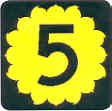# 5

This number is a prime.

Just showing those entries submitted by 'Burns': (Click here to show all)If you sum squares like 0^2 + 1^2 = 1, 0^2 + 1^2 + 2^2 = 5, 0^2 + 1^2 + 2^2 + 3^2 = 14, etc., it can be proven that the only prime you ever get for a sum is 5. [Burns]